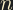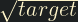## Problem statement

The prime factors of 13195 are 5, 7, 13 and 29.

What is the largest prime factor of the number 600851475143 ?

## Thoughts

This one will have to be solved using a loop and just checking if each prime divides. There is no known equation to find primes at certain positions like how we found theth Fibonacci number in problem 2

## Solution

We can use the standard prime factorisation approach of repeatedly dividing by successively higher primes, starting with two. We can limit our divisor toas there can't be two prime factors larger than the square root. By the time we get tothe dividend must already be the largest prime factor.

#include <math.h>
#include <stdio.h>

long target = 600851475143;

int main()
{
int i = 2;
while (pow(i, 2) < target) {
while (target % i == 0) {
target = target / i;
}
i++;
}
printf("%ld", target);
}